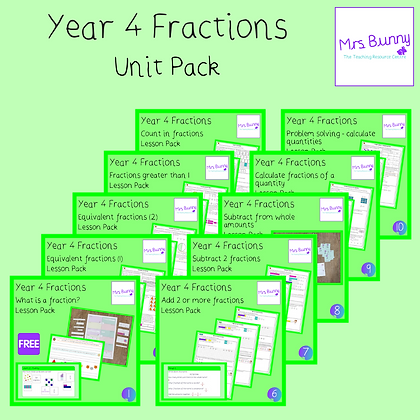# Year 4 Fractions Unit Pack

£13.50Price
`PREMIUM MEMBERS CLICK HERE TO DOWNLOAD. This pack contains all lessons and associated resources for the Year 4 Fractions unit of work. This includes lesson plans, worksheets and teaching presentations.	What is a fraction?	Equivalent fractions (1)	Equivalent fractions (2)	Fractions greater than 1	Count in fractions	Add 2 or more fractions	Subtract 2 fractions	Subtract from whole amounts	Calculate fractions of a quantity	Problem solving – calculate quantities`